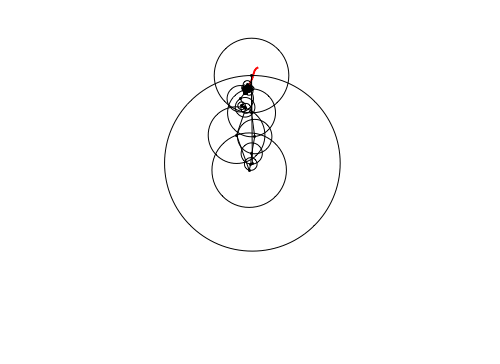# Wooster Epicycles

A vector is the sum of its components, a mechanical vibration is a combination of its normal mode motions, a quantum state is a superposition of its eigenstates, and any “nice” function is a Fourier sum of real or complex sinusoids, $e^{i \varphi} = \cos \varphi + i \sin \varphi$.

The animation below traces the Wooster W in epicycles of 100 circles-moving-on-circles in the complex plane. Algebraically, the trace is a complex discrete Fourier series $\sum c_n e^{i n \omega t} =\sum r_n e^{i (n\omega t + \theta_n)}$, where $r_n$ are the circle radii, $\theta_n$ are carefully chosen phase shifts, $\omega$ is the fundamental angular frequency, and $t$ is time.Using Fourier analysis, any reasonable path can be traversed by a moon orbiting a moon orbiting a moon orbiting … a planet orbiting a star